# Drawing Lines

 I l @ ve RuBoard

Flash MX contains a simple set of drawing commands that allow you to create a wide variety of graphics from scratch. You can draw straight lines, curves, and even filled areas.

#### Drawing Straight Lines

Drawing a line with ActionScript is easy. First, you need to define the line style. The lineStyle command takes three parameters: the line thickness , color, and alpha. The color is expressed as an ActionScript hexadecimal number. So 0x000000 is black, 0xFFFFFF is white, and so on. The alpha parameter determines a line's transparency. A value of 100 makes an opaque line, whereas a value of 50 makes a line that is faded 50 percent, and graphics behind it can be seen.

` lineStyle(3,0x000000,100); `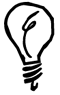If you use the line size of 0, you get a hairline . A hairline is a thin line that remains 1 pixel wide even if the line is scaled up. This is in contrast to a 1-pixel line that gets thicker as the scale of the movie increases .

After you have set the line style, you use lineTo and moveTo to draw. Imagine an invisible pen on the screen. You can direct its tip to move around on the screen. As it moves, you can tell it to draw on the screen, or just move without drawing.

The moveTo command moves the tip of the drawing pen to a location on the screen. The lineTo command moves the pen from its current location to another location and leaves a trail. Here is some code that draws a line from 275,200 to 300,225:

` moveTo(275,200); lineTo(300,225); `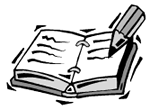If you do not use a moveTo command before you use a lineTo command, the first line will be drawn from point 0,0 to the point specified in the lineTo command.

Here is a short program that draws 500 lines from random points on the stage. You can see its result in Figure 24.1.

##### Figure 24.1. These random lines were created by ActionScript.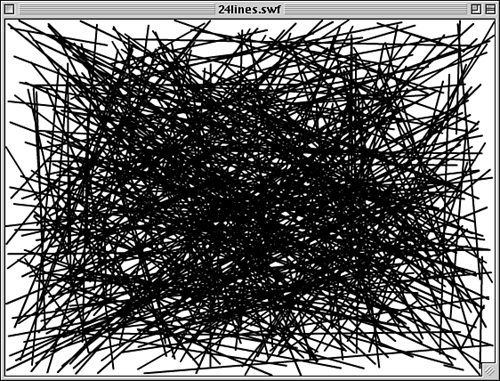` // set line style lineStyle(2,0x000000,100); // draw 500 lines for(var i=0;i<500;i++) {     // pick random start point     x1 = Math.random()*550;     y1 = Math.random()*400;     // pick random end point     x2 = Math.random()*550;     y2 = Math.random()*400;     // move to start point     moveTo(x1,y1);     // draw to end point     lineTo(x2,y2); } `

Check out the example movie 24lines.fla. You can play with the line size, color, and alpha. When you set the alpha to 50, it gives the impression that the lines are laying on top of and under each other. Check out 24coloredlines.fla to see a variation where the lines all draw as different colors.

Here is a simple script that draws a crossed pattern on the screen. It creates diagonal lines in each direction all the way across the screen.

` // set line style lineStyle(2,0x999999,100); for(var x=-400;x<550;x+=10) {     // draw diagonal strip from left to right     moveTo(x,0);     lineTo(x+400,400);     // draw opposite strip     moveTo(550-x,0);     lineTo(550-x-400,400); } `

Figure 24.2 shows the results of this script. One interesting note is that the movie 24patterns.fla takes up only 186 bytes as an .swf file. However, if you were to create the lines by hand using Flash's drawing tools and make a movie with those lines, the file would be 4,179 bytes. So you can actually save file space by drawing patterns with ActionScript rather than including them in the Flash movie as normal graphics.

##### Figure 24.2. This pattern was created with ActionScript.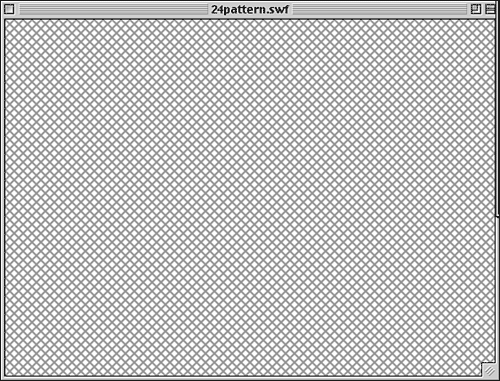#### Drawing Curves

Whereas drawing lines is easy and straightforward, drawing curves is more of an art than a science. In addition to giving the destination position where the curved line draws to, you also give a control point. This control point directs the curve of the line.

For instance, the following code draws a curve from 150,200 to 400,200. The second pair of numbers in the curveTo command gives the destination. The first pair is the control point. In this case, the control point is at 275,275, which is a little below the center of the line. This causes the line to curve down toward the control point.

` lineStyle(3,0x000000,100); moveTo(150,200); curveTo(275,275,400,200); `

Figure 24.3 shows the result. There are horizontal grid lines at 200 and 275, and vertical grid lines at 150, 275, and 400. You can see that the line starts at 150,200 and ends at 400,200. In between, it curves out to 275,275 but never reaches that point. The example movie 24curve.fla includes the grid lines that are drawn with lineTo commands.

##### Figure 24.3. Ever see ActionScript smile at you?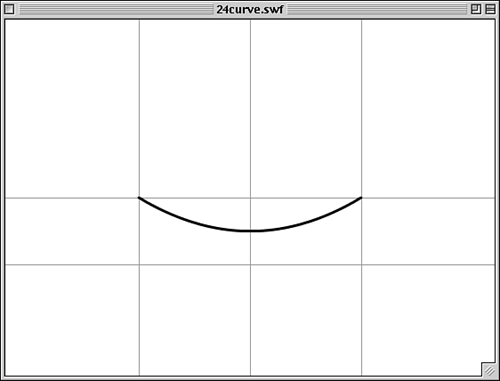Although you can control exactly where the curve starts and ends, you only have vague control over where the curve goes in between. However, by playing around with different values, you can get close to drawing what you want.

This code creates a square shape. The lines are drawn with the curveTo command, but the control points are placed right between the points so that the lines have no curve to them at all.

` lineStyle( 1, 0x0000FF, 100 ); moveTo(200,200); curveTo(250,200,300,200); curveTo(300,250,300,300); curveTo(250,300,200,300); curveTo(200,250,200,200); `

In Figure 24.4, you can see that the lines have no curve. The larger dots point out where the control points are located.

##### Figure 24.4. These curves form a square with straight sides.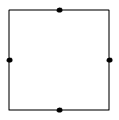Now, if we move the control points farther away from each side, we can bulge out the sides of the square to make it resemble a circle:

` var bend = 42; moveTo(200,200); curveTo(250,200-bend,300,200); curveTo(300+bend,250,300,300); curveTo(250,300+bend,200,300); curveTo(200-bend,250,200,200); `

I used the variable bend so that the control point would be moved away from each side by the same amount. The top of the square's control point is moved up, the left side's control point is moved to the left, and so on. I can adjust how far the control point is from the original side by just changing the value of bend in one place. Doing so, I was able to experiment and determine that 42 is the value that made it look best. Figure 24.5 shows this version of the drawing, complete with marks for the four control points.

##### Figure 24.5. This circle was drawn with only four curves. The dots show the curve's control points.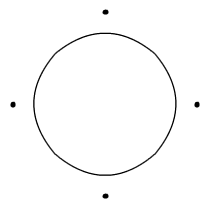The sample movie 24curvedcircle.fla contains both examples from Figures 24.4 and 24.5.Although drawing a circle is a great way to start playing with the curveTo command, the curveTo command is not the best way to draw a circle. Instead, you can get better results by drawing short lines from points along the circumference of a circle using the trigonometry from Hour 11, "Working with Numbers." You can see an example in the file 24bettercircle.fla.

#### Drawing Filled Areas

To draw a filled area, first you have to plan to draw a series of lines or curves that form a closed shape. Then all you need to do is issue a beginFill command before you start drawing and an endFill command when you are finished.

The beginFill command takes two parameters: the color of the fill and the alpha of the fill. In this example, a box is made out of a 3-pixel thick black line. The fill is red.

` lineStyle( 3, 0x000000, 100 ); beginFill( 0xFF0000 ); moveTo(175,100); lineTo(375,100); lineTo(375,300); lineTo(175,300); lineTo(175,100); endFill(); `

When an area is crossed by the line twice, it remains unfilled instead of being filled. This can create interesting gaps in your filled area. For instance, the following code draws a star with five points. The area at the center of the star remains unfilled. Look at Figure 24.6 to see the result.

##### Figure 24.6. The area double-crossed by the lines is not filled.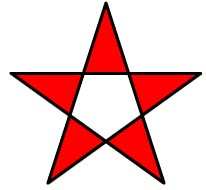` lineStyle(3,0x000000,100 ); beginFill(0xFF0000); moveTo(250,50); lineTo(308,230); lineTo(155,120); lineTo(345,120); lineTo(192,230); lineTo(250,50); endFill(); `There is a better way to make a star. The example movie 24betterstar.fla not only makes stars based on trigonometry but also allows you to set the number of points in the star at the start of the script. Create 5-, 7-, 11-, or 123-pointed stars.

 I l @ ve RuBoard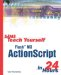Sams Teach Yourself Flash MX ActionScript in 24 Hours
ISBN: 0672323850
EAN: 2147483647
Year: 2002
Pages: 272